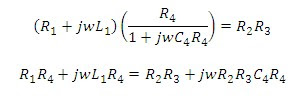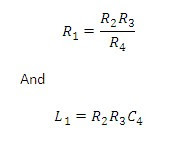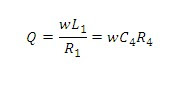# Maxwell’s inductance capacitance bridge

## Maxwell’s inductance capacitance bridge:In Maxwell’s inductance capacitance bridge the value of inductance is measured by the comparison with standard variable capacitance. The connection for Maxwell’s inductance capacitance bridge is shown in figure below.

Let

L1=unknown inductance,

R1=effective resistance of inductor L1,

R2, R3, R4=known noninductive resistances,

C4=variable standard capacitor.

And writing the equation for balanceSeparating the real and imaginary terms, we haveThus we have two variables R4 and C4 which appear in one of the two balance equations and hence the two equations are independent.

The expression for Q factor### Advantages of Maxwell’s inductance capacitance bridge:

1)      The two balance equations are independent if we choose R4 and C4 as variable elements.

2)      The frequency does not appear in any of the two equations.

3)      This bridge yields simple expressions for L1 and R1 in terms of known bridge elements.

### Disadvantages of Maxwell’s inductance capacitance bridge:

• This bridge requires a variable standard capacitor which may be very expensive if calibrated to the high degree of accuracy. Therefore sometimes a fixed standard capacitor is used, either because a variable capacitor is not available or because fixed capacitors have a higher degree of accuracy and are less expensive than the various ones. The balance adjustments are then done by:

a)      Either varying R2 and R4 and since R2 appears in both the balance equations, the balance adjustments become difficult; or

b)      Putting an additional resistance in series with the inductance under measurement and then varying this resistance and R4.

• The bridge is limited to the measurement of low Q coils (1<Q<10). it is clear from the Q factor equation that the measurement of high Q coils demands a large value of resistance R4, perhaps 10^5 or 10^6 O. The resistance boxes of such high values are very expensive. thus for values of  Q>10, the Maxwell's bridge is unsuitable.

1.2.